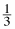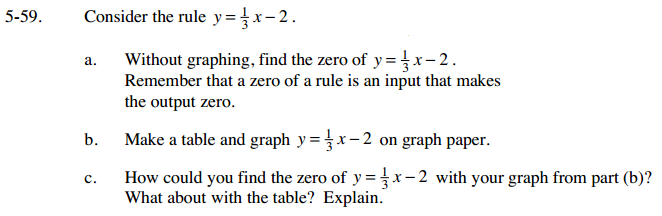### Home > AC > Chapter 5 > Lesson 5.1.6 > Problem5-59

5-59.
1. Consider the rule y =x − 2 . 5-59 HW eTool (Desmos). Homework Help ✎

1. Without graphing, find the zero of y =x − 2. Remember that a zero of a rule is an input that makes the output zero.

2. Make a table and graph y =x − 2 on graph paper.

3. How could you find the zero of y =x − 2 with your graph from part (b)? What about with the table? Explain.Set the equation equal to zero, since your output would be zero, and then solve for x.

Find the point where y = 0.